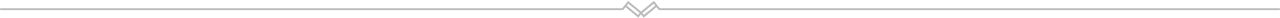# Using PHP date_diff() with and end date before the start date

How to use the PHP date_diff() function if you need to work with dates that will result in negative answers.Usually, you will calculate the difference between dates where the Start Date is older than the End Date.

You might come across instances where the Start Date is younger than the End Date with a negative result.

Dates, in general, is not easy to work with and in most cases need some magic to make it work properly. So without a doubt, the PHP function date_diff() is a life savour in many ways.

In this case study, I will use the current date as the Start Date and an End Date retrieved from a database. Under “normal” circumstances the second date will be later than the current date.

## Using PHP date_diff()

```<?php
\$now_date = date("Y-m-d");// set the current date
\$date1=date_create(\$now_date);// Create a DateTime object
\$date2=date_create(\$next_date);// Create a DateTime object
\$diff=date_diff(\$date1,\$date2);// Determine the difference between the dates and set a variable \$diff
echo ' <strong>Expires in '.\$diff->format("%a days").'days</strong>';// display the date in your app
?>```

The above output will look something like this: Expires in 242 days

If the End Date is older than the current date, the result will still look like this: Expires in 122 days. No indication that the result should be a negative value

Changing the last line of code to the following will fix that error

`echo ' <strong>Expires in '.\$diff->format("%R%a days").'days</strong>';// display the date in your app`

The result will look something like this: Expires in +242 days and Expires in -122 days. Now the user can see if the dates are in the feature or past

### User Friendly PHP date_diff()

The above can work but it is, in my opinion, not user-friendly and need some more work

The solution lies in a parameter of the PHP DateInterval class

Update your code as follows

```<?php
\$now_date = date("Y-m-d");// set the current date
\$date1=date_create(\$now_date);// Create a DateTime object
\$date2=date_create(\$next_date);// Create a DateTime object
\$diff=date_diff(\$date1,\$date2);// Determine the difference between the dates and set a variable \$diff
//echo ' <strong>Expires in '.\$diff->format("%R%a days").'days</strong>';// comment out this line

// add the following and format your output properly
if (\$diff->invert == 1)// Is 1 if the interval represents a negative time period and 0 otherwise
{
echo '<strong>Expired'.\$diff->format("%a days").'ago</strong>';
}else
{
echo ' <strong>Expire in '.\$diff->format("%a days").'</strong>';
}
?>```

The output from above will now look like this:

Expires in 242 days or Expired 242 days ago

Hope this helps someone...

Written by:  - 1 Nov, 2019